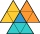# Cosine + The Law of Sines - math problems

#### Number of problems found: 7

• Calculate triangleIn the triangle ABC, calculate the sizes of all heights, angles, perimeters and its area, if given a-40cm, b-57cm, c-59cm
• Triangle's centroidIn the triangle ABC the given lengths of its medians tc = 9, ta = 6. Let T be the intersection of the medians (triangle's centroid) and point is S the center of the side BC. The magnitude of the CTS angle is 60°. Calculate the length of the BC side to 2 d
• Viewing angleThe observer sees a straight fence 60 m long at a viewing angle of 30°. It is 102 m away from one end of the enclosure. How far is the observer from the other end of the enclosure?
• The aspect ratioThe aspect ratio of the rectangular triangle is 13: 12: 5. Calculate the internal angles of the triangle.
• Children playgroundThe playground has a trapezoid shape, and the parallel sides have a length of 36 m and 21 m. The remaining two sides are 14 m long and 16 m long. Find the size of the inner trapezoid angles.
• ABCDAC= 40cm , angle DAB=38 , angle DCB=58 , angle DBC=90 , DB is perpendicular on AC , find BD and AD
• Inner anglesThe inner angles of the triangle are 30°, 45° and 105° and its longest side is 10 cm. Calculate the length of the shortest side, write the result in cm up to two decimal places.

We apologize, but in this category are not a lot of examples.
Do you have an exciting math question or word problem that you can't solve? Ask a question or post a math problem, and we can try to solve it.

We will send a solution to your e-mail address. Solved examples are also published here. Please enter the e-mail correctly and check whether you don't have a full mailbox.

Please do not submit problems from current active competitions such as Mathematical Olympiad, correspondence seminars etc...

Cosine - math problems. The Law of Sines - math problems.